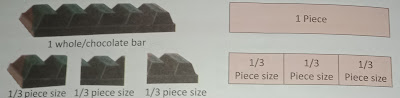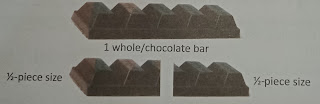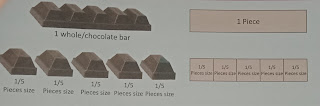# Lesson Planning of Division of Fraction

Lesson Planning of Division of Fraction

Subject Mathematics

Students` Learning Outcomes

• Divide fraction by a whole number
• Divide a whole number by a fraction
• Divide a fraction by another fraction (proper, improper and mixed fractions)

Information for Teachers

• You know how to call the numbers in division?
• To divide 2 fractions multiply the divided by the reciprocal of the divisor.• Reciprocal of a fraction is [1÷fraction] and it turns the fraction upside down
• Encourage students to help each other in learning these skills.• While teaching the lesson, the teacher should also consult with textbook at all steps where and when applicable.

Material / Resources

Writing board, chalk/marker, duster, chart paper, textbook

Introduction

• Tell story problems that involve division with whole number on the board.
• Maria has a dozen biscuits. These biscuits have to be shared equally with 6 of her family members. How many biscuits will each of her family member get/?
• Salma wants to get jogging in 2 hours; she can jog a total of 8 miles. How many miles cn she jog in one hour?
• Mother made 36 chocolate chip cookies. How many jars holding 9 cookies each can she fill?
• One day Fiaz collected 60 eggs from his hens on the farm. How many boxes holding 6 eggs each can he fill?
• Ask the students to use any strategy they choose to solve these problems and to show their work.
• Ask the following questions: as;
• What kind of problems are these, Addition, Subtraction, Multiplication, or division? (Expected answer would be as; Division)
• Tell students that today we will divide fractions the way we have done multiplication of fractions.

Development

 Activity 1 Write / tell a story problem involving the division of whole numbers by fractions on the board. I had one chocolate bar. I divided it by one-half and give an equal-sized piece to each of my friends. How many one-half-sized pieces of chocolate make up that one chocolate bar? Explain with following questions: as; How many chocolate bars did we have? How did we want to divide each chocolate bar? After we divided it, how many pieces of chocolate did we get? Was the size of the whole chocolate bar(s) different from the desired pieces sizes? How can we compare size of the whole chocolate bar with size of th pieces? Demonstrate the concept with a visual representation.Count total ½-pieces size in 1 whole / chocolate bar. Have the students work with a partner? Have the students try to solve the remaining problems, such as dividing the one whole chocolate bar into three pieces and five pieces? The representation of chocolate bar would be as follows: as;Ask: Do you see a pattern or relationship in each problem? What is the pattern or relationship in each problem? Have the students share and discuss their answers? Share the following rules with the students: as; Size of chocolate = 1 whole number of pieces required = 5 size of each piece = 1 ÷ 5 size of each piece = 1 x (1 / 5) When one whole is divided into five parts each part is called “one fifth or 1 / 5” Since there are 5 pieces, we can say that: One whole chocolate bar = 5 pieces of one-fifth size. One whole chocolate bar = 5 pieces x 1 / 5 pieces One whole piece of chocolate = one whole piece of chocolate.

 Activity 2 Explain following steps with the help of example, as; To divide 2 fractions multiply the dividend by the reciprocal of the divisor. Reciprocal of a fraction is [1÷ fraction] and it turns the fraction upside down. you can do this by following few simple steps: Change all mixed fractions (if any) to improper fractions. Turn the second fraction (divisor) upside down. Change the divide sign to multiply sign and multiply the fractions.Write few more examples on the board and solve with the help of the students. Refer students in pairs to textbook exercise. Students will solve their work individually. After completing work they will swap their notebooks for peer checking.

Sum up / Conclusion
• Reciprocal of a fraction is 1 fraction, or simply invert the fraction.
• To multiply;
• Change mixed number fractions into improper fraction.
• Take invert of the second fraction
• Multiply the fractions and convert into mixed number fraction if answer comes in improper fractions.

Assessment

• Divide students in groups and provide them two dice in each group.
• Ask students to roll the dice and make a fraction with numbers they get.
• Again roll and make another fraction. Divide both fractions and get the answer.
• Allocate 10 minutes and tell them that the group with maximum questions within the time will win the round’